# Channel with a finite number of states

(diff) ← Older revision | Latest revision (diff) | Newer revision → (diff)

finite-state channel

A communication channel for which the statistical properties of the output signal at a timeare determined by the input signal at this moment and the state of the channel at the previous moment, and where the set of possible states of the channel is finite. A channel with a finite number of states can also be defined by a finite probabilistic automaton (cf. Automaton, probabilistic). Below a rigorous definition is given of a discrete-time homogeneous channel with a finite number of states and with finite spaces of values,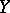and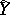, for the components of the input and output signals. Suppose that functions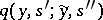,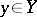,,, are given, whereis a finite set, called the set of states of the channel, as well as a probability distribution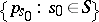. Intuitively, the functiondefines the conditional probability that at a time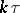the signal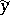appears at the output and the channel goes over to the state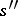under the condition that the signal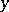was transmitted and that at the previous moment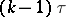the channel was in state. The distributioncan be regarded as the probability distribution of the initial state of the channel (that is, the state of the channel at the initial moment). Let the functions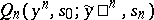be recursively defined by the formulas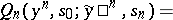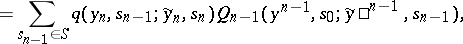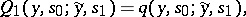where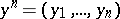,,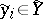,,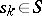,. Let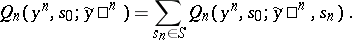Then the transition function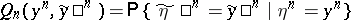of segments of lengthof the channel with a finite number of states, for any, is, by definition, equal to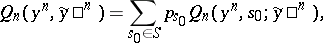whereand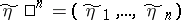are the segments of lengthof the input and the output of the channel.

For references see ,

in the article Communication channel.

How to Cite This Entry:
Channel with a finite number of states. Encyclopedia of Mathematics. URL: http://encyclopediaofmath.org/index.php?title=Channel_with_a_finite_number_of_states&oldid=18715
This article was adapted from an original article by R.L. DobrushinV.V. Prelov (originator), which appeared in Encyclopedia of Mathematics - ISBN 1402006098. See original article